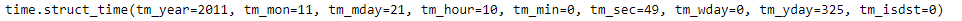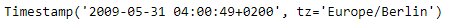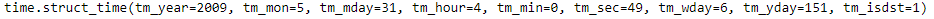# Python | Pandas Timestamp.timetuple

Python is a great language for doing data analysis, primarily because of the fantastic ecosystem of data-centric python packages. Pandas is one of those packages and makes importing and analyzing data much easier.

Pandas` Timestamp.timetuple()` function return a time tuple for the given Timestamp object. The returned tuple contains values ranging from year, month to hours and seconds. The time tuple is compatible with time.localtime().

Syntax :Timestamp.timetuple()

Parameters : None

Return : time tuple

Example #1: Use `Timestamp.timetuple()` function to return a time tuple for the given Timestamp object.

 `# importing pandas as pd ` `import` `pandas as pd ` ` `  `# Create the Timestamp object ` `ts ``=` `pd.Timestamp(year ``=` `2011``,  month ``=` `11``, day ``=` `21``,  ` `                  ``hour ``=` `10``, second ``=` `49``, tz ``=` `'US/Central'``)  ` ` `  `# Print the Timestamp object ` `print``(ts) `

Output :Now we will use the `Timestamp.timetuple()` function to return a time tuple.

 `# return time tuple ` `ts.timetuple() `

Output :As we can see in the output, the `Timestamp.timetuple()` function has returned a tuple for the given Timestamp object which contains values like year, month, day etc.

Example #2: Use `Timestamp.timetuple()` function to return a time tuple for the given Timestamp object.

 `# importing pandas as pd ` `import` `pandas as pd ` ` `  `# Create the Timestamp object ` `ts ``=` `pd.Timestamp(year ``=` `2009``, month ``=` `5``, day ``=` `31``,  ` `                  ``hour ``=` `4``, second ``=` `49``, tz ``=` `'Europe/Berlin'``) ` ` `  `# Print the Timestamp object ` `print``(ts) `

Output :Now we will use the `Timestamp.timetuple()` function to return a time tuple.

 `# return time tuple ` `ts.timetuple() `

Output :As we can see in the output, the `Timestamp.timetuple()` function has returned a tuple for the given Timestamp object which contains values like year, month, day etc.

My Personal Notes arrow_drop_upCheck out this Author's contributed articles.

If you like GeeksforGeeks and would like to contribute, you can also write an article using contribute.geeksforgeeks.org or mail your article to contribute@geeksforgeeks.org. See your article appearing on the GeeksforGeeks main page and help other Geeks.

Please Improve this article if you find anything incorrect by clicking on the "Improve Article" button below.

Article Tags :

Be the First to upvote.

Please write to us at contribute@geeksforgeeks.org to report any issue with the above content.